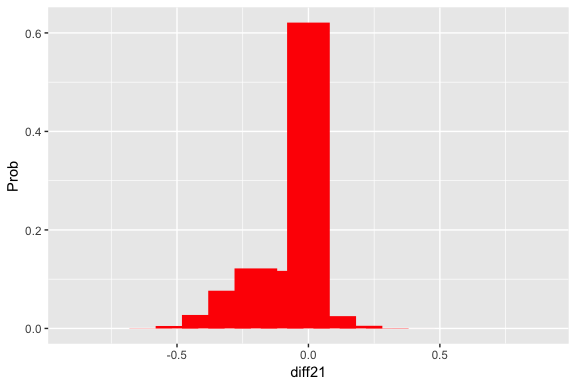# Two_Proportions

The TeachBayes package has several functions to facilitate working with a discrete prior for two proportions.

library(TeachBayes)

### Uniform Prior

Start with a uniform prior on (p1, p2), where each proportion takes on values .05, .15, …, .95.

values <- seq(.05, .95, by=.1)
prior <- prior.two.parameters(values, values)

Construct a graph of this distribution.

draw_two_p(prior)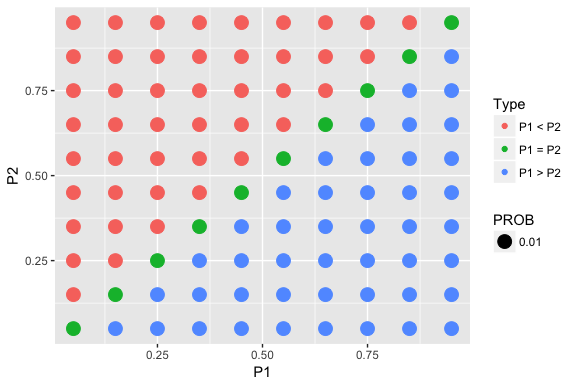This finds the probability distribution of the difference in proportions p1 - p2.

prob_plot(two_p_summarize(prior))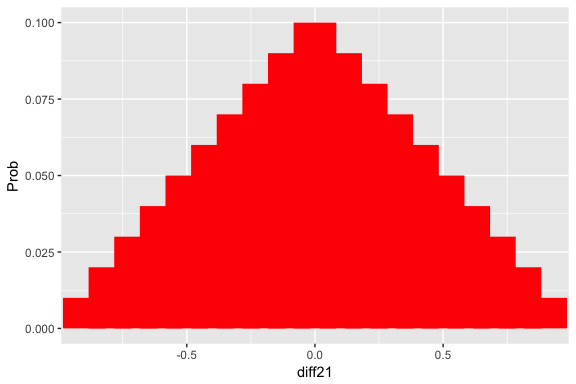Collect some data from two binomial samples.

y1n1 <- c(10, 20)
y2n2 <- c(8, 24)

Update (find posterior):

post <- two_p_update(prior, y1n1, y2n2)

Graph and summarize:

draw_two_p(post)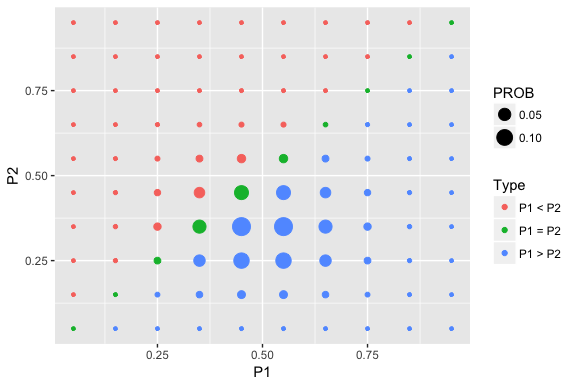prob_plot(two_p_summarize(post))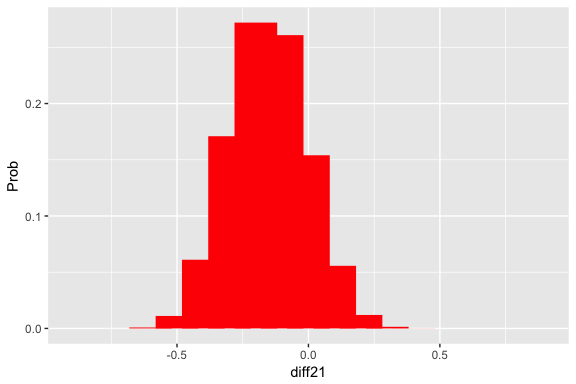### Testing Prior

prior <- testing_prior(.05, .95, 10, .5)

Construct a graph of this distribution and summarize.

draw_two_p(prior)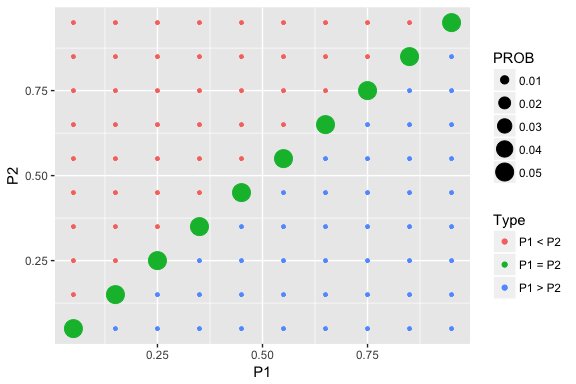prob_plot(two_p_summarize(prior))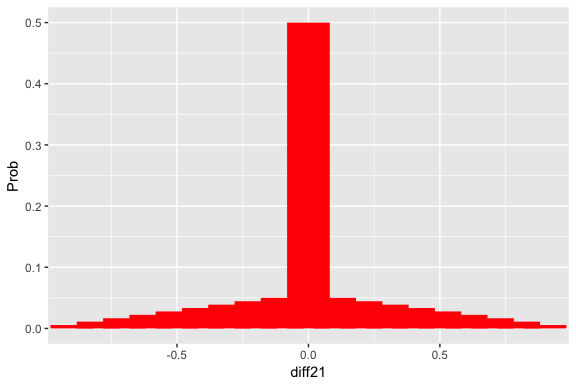Collect some data from two binomial samples.

y1n1 <- c(10, 20)
y2n2 <- c(8, 24)

Update (find posterior):

post <- two_p_update(prior, y1n1, y2n2)

Graph and summarize:

draw_two_p(post)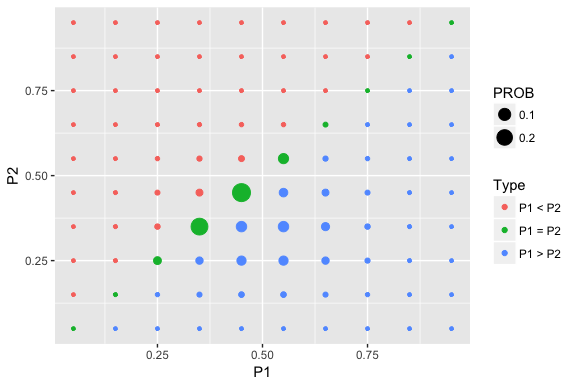prob_plot(two_p_summarize(post))